## Monday, July 11, 2011

### Transmission Lines and the Local Loop

I know this post gets a little mathematical. Try and think of the math in simple terms - in the examples below we're dealing with some basic division:

That's numerator (top number) divided by denominator (bottom number) in the equation.

If the numerator is large compared to the denominator then the answer is going to be relatively large (think big number divided by small number gives big number answer and remember...... everything is relative :) ). And vice versa - if the numerator is small compared to the denominator then the answer is going to be small ((think small number divided by big number gives small number answer).

This should help to understand the examples below.

----------------------------------

In my last post I wrote about the local loop - that pair of copper telephone wires most of us still have coming into out homes.These wires have been used for voice in some places for close to 100 years and now, using DSL technologies, to deliver voice and data. AT&T UVerse is even using the local loop to deliver triple play services - voice, video and data. In this post, let's take a little close look transmission lines.

The local telephone loop (also referred to as the subscriber loop) is the dedicated copper wire twisted pair connecting a telephone company Central Office (CO) in a locality to a customer home or business. The loop resistance is critical in the local loop and phone companies have had to “tune” the loop to transmit high-quality voice. Typically, companies have used 19 gauge (1.25 decibels [dB] attenuation per mile) to 26 gauge (3 dB attenuation per mile) copper wire for the local loop. The average customer local loop is about 2 miles and attenuation on this loop is ideally kept below 8 dB.

We can look at a typical transmission line model and use it to represent a subscriber loop:
Transmission Line Model

We can see that the inductance (L), resistances (R for series resistance and S for shunt resistance), and capacitance (C) are distributed throughout the model. We can also show that these values cause signal loss and distortion.  A local loop copper wire pair effectively forms a capacitance since you have two conductors (copper wire) separated by an insulator (wire insulation). Shunt or mutual capacitive reactance is independent of wire gauge and local loop wire pairs designed for voice have a capacitance value of about .083 μF/mile.

In addition to local loop cable, copper cables designed for higher frequencies like those used for T carrier systems are designed to provide a capacitance of .066 μF/mile.

Two Wires Separated by Insulation Forming a Capacitance

Capacitive reactance is basically the resistance of a capacitance and it changes with frequency.   The formula for capacitive reactance is: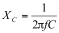The units for capacitive reactance are Ohms (Ω). Looking at the formula you can see as frequency increases the denominator gets larger so the capacitive reactance drops. On long local loops (3 miles and greater) shunt capacitance values increase to the point where significant signal leakage occurs at frequencies greater than 1000 Hz. If you look at the formula, you realize the higher the frequency the greater the leakage loss. Let’s look at some examples:

Example A

A local loop is 1 mile long. Calculate the capacitive reactance for the loop at 2KHz.

Solution:
Usingf = 2 KHz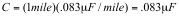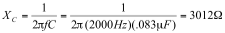Example B

This same local loop is extended to 3 miles. Calculate the new capacitive reactance for the loop at 2KHz

Solution:
Usingf = 2 KHz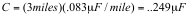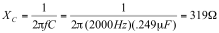In the example you can see that, by increasing the length of the loop by two miles, shunt capacitance drops by a factor close to 10.

In addition to length, higher frequencies also cause shunt capacitance reactance to increase.

Example C

Let’s increase the frequency in Example B to 3KHz and calculate the capacitive reactance of the local loop.

Solution:

Usingf = 3 KHz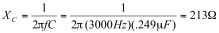Example D

Let’s now decrease the frequency to 1KHz and calculate the capacitive reactance of the local loop.

Solution:

Usingf = 1 KHz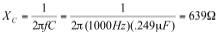Now consider a voice conversation on the Example C local loop. We know the frequency range of the local loop is approximately 300 Hz to 3300 Hz. We know the human voice can produce frequencies of both 3KHz and 1KHz and the average ear can hear these frequencies. At 1 KHz we have a shunt capacitive reactance of 639Ω and  at 3 KHz we have a shunt capacitive reactance of 213Ω. You can see more signal is lost due to capacitive shunting at the higher frequencies than at the lower frequencies. When it comes to voice - the listener will notice these differences – the lower frequencies in a voice conversation will appear louder than the higher frequencies in a conversation.

Over 100 years ago telephone companies figured out they could "load" a transmission line with inductors (loading coils) to reduce the effects of capacitive reactance. I'll discuss loading coils in a future post.